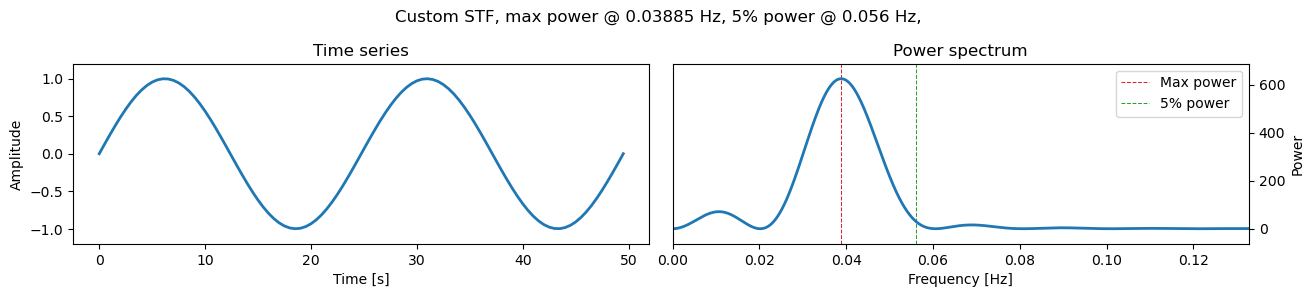Version:This tutorial is presented as Python code running inside a Jupyter Notebook, the recommended way to use Salvus. To run it yourself you can copy/type each individual cell or directly download the full notebook, including all required files.# Custom Source Time Functions

Copy
%matplotlib inline
%config Completer.use_jedi = False
# Standard Python packages
import os
import pathlib

# Third-party imports.
import numpy as np
import matplotlib.pyplot as plt
import pyasdf

# Salvus packages
import salvus.flow.api
import salvus.flow.simple_config as sc
from salvus.mesh.simple_mesh import basic_mesh

SALVUS_FLOW_SITE_NAME = os.environ.get("SITE_NAME", "local")

## Main message

Salvus offers a number of parameterized source time functions (STFs) - if that is not sufficient one can construct a custom one from a numpy array as illustrated in the following cell. Several rules apply:
• The array has to have the shape [npts, N] where npts the number of samples and N is the number of source components. The order can also be reversed in which case the array will automatically be transposed by SalvusFlow before the simulations are run.
• The spatial weights (f[_x, ...], m_[xx, yy, ...]) are always multiplied on top of the given STFs. Thus either normalize these or set the weights all to 1.0.
• For sources that have multiple components, e.g. vectorial or moment tensor sources, N is either equal to the number of independent source components (xy[z] in the vectorial case, m_xx, m_yy, ... in the tensor case) or equal to 1 in which case a copy of that array will be created before the weights are applied.
• The order of the given components must either be xy[z] in the vectorial case or adhering to the Voigt notation in the tensor case.
• Potentially specified rotation matrizes for the sources are applied after the weights and original STFs have been multiplied.
# A single array.
stf = sc.stf.Custom.from_array(
np.sin(np.linspace(0, 4 * np.pi, 100)),
sampling_rate_in_hertz=2.0,
start_time_in_seconds=0.0,
)
stf.plot()

# Combine with a source object to create a complete source object.
# Note the different weights will define the final source together
# with the STF.
src = sc.source.cartesian.VectorPoint2D(
x=10.0, y=0.0, fx=1e-2, fy=-2e-2, source_time_function=stf
)# It is also possible to specify a separate array for every components.
array = np.array(
[
1.5 * np.sin(np.linspace(0, 4 * np.pi, 100)),
-3.0 * np.sin(np.linspace(0, 4 * np.pi, 100)),
]
)

stf = sc.stf.Custom.from_array(
array, sampling_rate_in_hertz=2.0, start_time_in_seconds=-10.0
)
stf.plot()

# Note that in this case the weights should be set to 1.0
src = sc.source.cartesian.VectorPoint2D(
x=10.0, y=0.0, fx=1.0, fy=1.0, source_time_function=stf
)